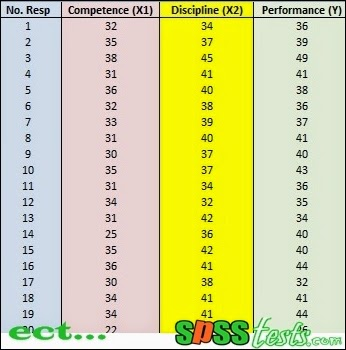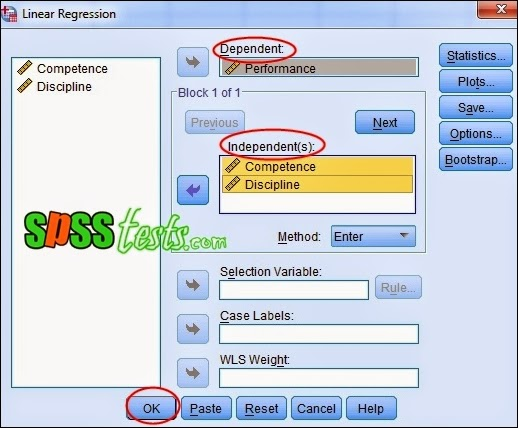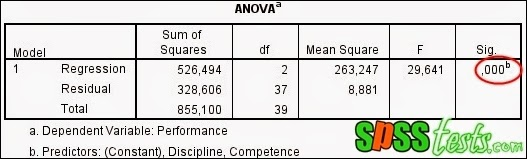# Method Multiple Linear Regression Analysis Using SPSS

Method Multiple Linear Regression Analysis Using SPSS | Multiple linear regression analysis to determine the effect of independent variables (there are more than one) to the dependent variable. To test multiple linear regression first necessary to test the classical assumption includes normality test, multicollinearity, and heteroscedasticity test. Classical assumption does this because independent variables studied amounted to more than one.

Decision-making process in Multiple Linear Regression Analysis
1. If the value of Significance <0.05, significant effect of independent variables on the dependent variable
2. If the value Signification> 0.05, then the independent variable has no significant effect on the dependent variable

Case in Multiple Linear Regression Analysis

A company manager wants to know whether there is an influence of Competence and Discipline of the Employee Performance. In this case the competence is X1 and X2 is the discipline, while the performance is Y. Furthermore, the manager collects data competence, Discipline, and Performance of 40 samples of employees. The research data as following picture.Step-by-Step Multiple Linear Regression Analysis Using SPSS

1. Turn on the SPSS program and select the Variable View. Furthermore, definition studies variables so that the results fit the picture below.2. Then, click the Data View, and enter the data competence, Discipline and Performance3. Next, from the SPSS menu click Analyze - Regression - linear4. Will display box Linear Regression, then enter Competence and Discipline to box Independent (s), then insert Performance into the box Dependent5. The last step click Ok, after which it will appear SPSS output, as follows

(Output Model Summary)(Output ANOVA)(Output Coefficients a)Interpretation of Results of Multiple Linear Regression Analysis Output

(Output Model Summary)
In this section display the value of R = 0.785 and the coefficient of determination (Rsquare) of 0.616. This suggests the notion that performance (Y) is influenced by 61.6% by Competence (X1) and Discipline (X2), while the rest (100% -61.6% = 38.4%) is explained by other causes.

(Output ANOVA)
In this section displayed a probability level of significance value of 0.000. Therefore the probability (0.000) is much smaller than 0.05, then the multiple regression models can be used to predict the performance of employees. Or in other words, Competence (X1) and Discipline (X2) simultaneously significant effect on employee performance (Y)

(Output Coefficients a)
In this section displayed significant value Competence (X1) of 0.013 <0.05, then the appropriate basis for decision making in the regression analysis can be concluded that the Competence (X1) partially significant effect on employee performance (Y). Thus, increasing the competence of a person it will also improve performance.

Meanwhile, the significance of Discipline (X2) of 0.001 <0.05, it can be concluded that the Discipline (X2) partially significant effect on employee performance (Y). Thus, increasing one's discipline will also improve performance.

[Keywords: How Multiple Linear Regression Analysis Using SPSS | Multiple Linear Regression Testing Using SPSS | Tutorial Multiple Linear Regression with SPSS Software Complete | Step-by-Step Multiple Linear Regression Analysis]
[Image: Data SPSS Version 21]
[Source: Summarized from various sources]

### 3 Responses to "Method Multiple Linear Regression Analysis Using SPSS"

1.1.2.Please I want a Dataset of 656 sample size. Topic emotions intelligence (self awareness, empathy, self esteem, social awareness) and academic Performance of students. Thank you